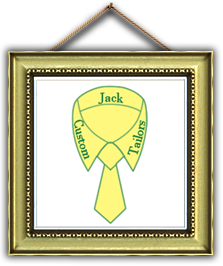•••••••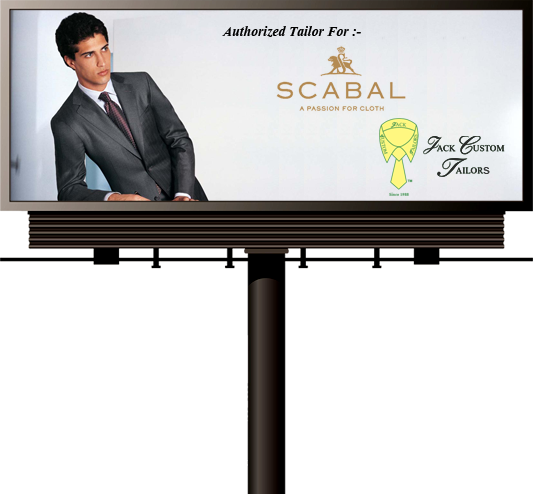•••••••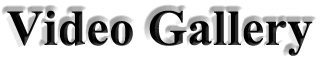x Closex Closex Closex Closex Closex Closex Closex Closex Closex Closex Closex Closex Closex Closex Closex Closex Closex Closex Closex Closex Close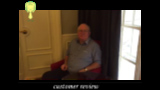x Closex Closex Closex Closex Closex Closex Closex Closex Closex Closex Closex Closex Closex Closex Closex Closex Closex Closex Closex Closex Closex Closex Closex Closex Closex Closex Closex Closex Closex Closex Closex Closex Closex Closex Closex Closex Closex Closex Closex Close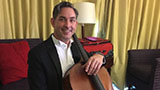x Close